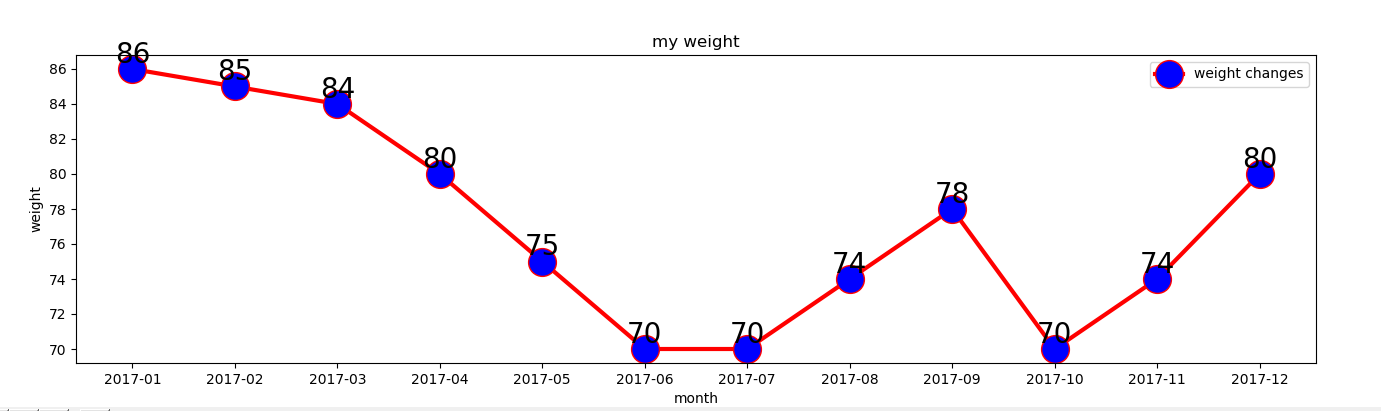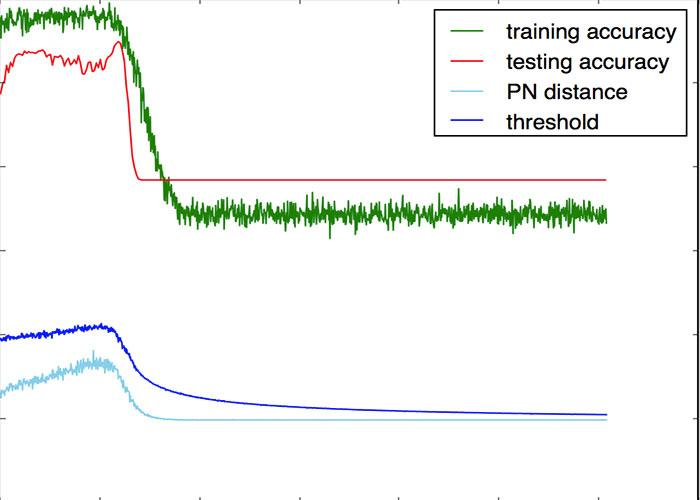• 1.5) # 蓝色的线 plt.plot(y.cumsum(),'ro') #离散的 plt.grid(True) plt.axis('tight') plt.xlabel('index') plt.ylabel('value') plt.title('A simple Plot') plt.show() 以上就是Python 绘制可视化折线图的详细...
1. 用 Numpy ndarray 作为数据传入 ply
import numpy as np
import matplotlib as mpl
import matplotlib.pyplot as plt
np.random.seed(1000)
y = np.random.standard_normal(10)
print "y = %s"% y
x = range(len(y))
print "x=%s"% x
plt.plot(y)
plt.show()2. 操纵坐标轴和增加网格及标签的函数
import numpy as np
import matplotlib as mpl
import matplotlib.pyplot as plt
np.random.seed(1000)
y = np.random.standard_normal(10)
plt.plot(y.cumsum())
plt.grid(True) ##增加格点
plt.axis('tight') # 坐标轴适应数据量 axis 设置坐标轴
plt.show()3. plt.xlim 和 plt.ylim 设置每个坐标轴的最小值和最大值
#!/etc/bin/python
#coding=utf-8
import numpy as np
import matplotlib as mpl
import matplotlib.pyplot as plt
np.random.seed(1000)
y = np.random.standard_normal(20)
plt.plot(y.cumsum())
plt.grid(True) ##增加格点
plt.xlim(-1,20)
plt.ylim(np.min(y.cumsum())- 1, np.max(y.cumsum()) + 1)
plt.show()4. 添加标题和标签 plt.title, plt.xlabe, plt.ylabel 离散点, 线
#!/etc/bin/python
#coding=utf-8
import numpy as np
import matplotlib as mpl
import matplotlib.pyplot as plt
np.random.seed(1000)
y = np.random.standard_normal(20)
plt.figure(figsize=(7,4)) #画布大小
plt.plot(y.cumsum(),'b',lw = 1.5) # 蓝色的线
plt.plot(y.cumsum(),'ro') #离散的点
plt.grid(True)
plt.axis('tight')
plt.xlabel('index')
plt.ylabel('value')
plt.title('A simple Plot')
plt.show()以上就是Python 绘制可视化折线图的详细内容，更多关于Python 绘制折线图的资料请关注python博客其它相关文章！
展开全文• 当我们获取完数据之后，一般来说数据可视化呈现的最基础图形就是：柱状图、水平条形图、折线图等等，在python的matplotlib库中分别可用bar、barh、plot函数来构建它们，再使用xticks与yticks（设置坐标轴刻度）、...
当我们获取完数据之后，一般来说数据可视化呈现的最基础图形就是：柱状图、水平条形图、折线图等等，在python的matplotlib库中分别可用bar、barh、plot函数来构建它们，再使用xticks与yticks（设置坐标轴刻度）、xlabel与ylabel（设置坐标轴标签）、title（标题）、legend（图例）、xlim与ylim（设置坐标轴数据范围）、grid（设置网格线）等命令来装饰图形，让它更明晰与美观，但是对于数据标签（即在图形中的每一个点或条形位置上标注出相应数值），却没有直接的命令导出。我们看很多python可视化做出的柱状图等等，在柱子数量不多的条件下，没有数据标签，只有光秃秃的一个个柱子，这样就使得图形缺了些什么。事实上，在python的matplotlib中有在图形中添加文字说明的命令——text，我们就可以利用这个命令，在图中每个单位要素中添加相应文字，来构造数字标签。
以本人专栏知乎专栏写的《大数据时代，哪些课程最受欢迎？》所爬取数据为例，经过numpy得到了不同课程类别下的平均学习人数，如图：v2-436583cccc6aaf0b95703e570b42cf45_b.png
plt.figure(figsize=(10,6))
#设置x轴柱子的个数
x=np.arange(14)+1 #课程品类数量已知为14，也可以用len(ppv3.index)
#设置y轴的数值，需将numbers列的数据先转化为数列，再转化为矩阵格式
y=np.array(list(ppv3['numbers']))
xticks1=list(ppv3.index) #构造不同课程类目的数列
#画出柱状图
plt.bar(x,y,width = 0.35,align='center',color = 'c',alpha=0.8)
#设置x轴的刻度，将构建的xticks代入，同时由于课程类目文字较多，在一块会比较拥挤和重叠，因此设置字体和对齐方式
plt.xticks(x,xticks1,size='small',rotation=30)
#x、y轴标签与图形标题
plt.xlabel('课程主题类别')
plt.ylabel('number')
plt.title('不同课程类别的平均学习人数')
**#设置数字标签**
**for a,b in zip(x,y):**
** plt.text(a, b+0.05, '%.0f' % b, ha='center', va= 'bottom',fontsize=7)**
#设置y轴的范围
plt.ylim(0,3700)
plt.show()
最终得到的图形为：不同类别学习人数.png
着重讲一下如何设置数字标签
#设置数字标签
for a,b in zip(x,y):
plt.text(a, b+0.05, '%.0f' % b, ha='center', va= 'bottom',fontsize=7)
首先，前边设置的x、y值其实就代表了不同柱子在图形中的位置（坐标），通过for循环找到每一个x、y值的相应坐标——a、b，再使用plt.text在对应位置添文字说明来生成相应的数字标签，而for循环也保证了每一个柱子都有标签。
其中，a, b+0.05表示在每一柱子对应x值、y值上方0.05处标注文字说明， '%.0f' % b,代表标注的文字，即每个柱子对应的y值， ha='center', va= 'bottom'代表horizontalalignment（水平对齐）、verticalalignment（垂直对齐）的方式，fontsize则是文字大小。
条形图、折线图也是如此设置，饼图则在pie命令中有数据标签的对应参数。对于累积柱状图、双轴柱状图则需要用两个for循环，同时通过a与b的不同加减来设置数据标签位置，比如在本文另一文章[《双十一预售战下的电商风云》]（https://zhuanlan.zhihu.com/p/23492721
） ，如图：电商平台商品评价.png
其命令为：电商代码.png
展开全文• 本文想说的是折线点的地方显示数据纵坐标数据 利用：plt.text() 方法 废话不多说，直接上代码： # encoding=utf-8 import matplotlib.pyplot as plt # 月份 x1 = ['2017-01', '2017-02', '2017-03', '2017-04...
本文想说的是折线点的地方显示数据纵坐标数据
利用：plt.text() 方法
废话不多说，直接上代码：

# encoding=utf-8
import matplotlib.pyplot as plt

# 月份
x1 = ['2017-01', '2017-02', '2017-03', '2017-04', '2017-05', '2017-06', '2017-07', '2017-08',
'2017-09', '2017-10', '2017-11', '2017-12']

# 体重
y1 = [86, 85, 84, 80, 75, 70, 70, 74, 78, 70, 74, 80]

# 设置画布大小
plt.figure(figsize=(16, 4))

# 标题
plt.title("my weight")

# 数据
plt.plot(x1, y1, label='weight changes', linewidth=3, color='r', marker='o',
markerfacecolor='blue', markersize=20)

# 横坐标描述
plt.xlabel('month')

# 纵坐标描述
plt.ylabel('weight')

# 设置数字标签
for a, b in zip(x1, y1):
plt.text(a, b, b, ha='center', va='bottom', fontsize=20)

plt.legend()
plt.show()

效果：展开全文matplotlib
• 今天小编就为大家分享一篇修改python plot折线图的坐标轴刻度方法，具有很好的参考价值，希望对大家有所帮助。一起跟随小编过来看看吧
• python画折线图利用的是matplotlib.pyplot.plot的工具来绘制折线图，这里先给出一个段代码和结果图：# -*- coding: UTF-8 -*-import numpy as npimport matplotlib as mplimport matplotlib.pyplot as plt#这里导入...python画折线图利用的是matplotlib.pyplot.plot的工具来绘制折线图，这里先给出一个段代码和结果图：# -*- coding: UTF-8 -*-
import numpy as np
import matplotlib as mpl
import matplotlib.pyplot as plt
#这里导入你自己的数据
#......
#......
#x_axix，train_pn_dis这些都是长度相同的list()
#开始画图
sub_axix = filter(lambda x:x%200 == 0, x_axix)
plt.title('Result Analysis')
plt.plot(x_axix, train_acys, color='green', label='training accuracy')
plt.plot(sub_axix, test_acys, color='red', label='testing accuracy')
plt.plot(x_axix, train_pn_dis, color='skyblue', label='PN distance')
plt.plot(x_axix, thresholds, color='blue', label='threshold')
plt.legend() # 显示图例
plt.xlabel('iteration times')
plt.ylabel('rate')
plt.show()
#python 一个折线图绘制多个曲线
结果如下这里谈谈matplotlib.pyplot.plot()的使用方法，然后我说下可能用到的一些参数，参数可选的内容我就不一一展开了，需要的可自行查询官方文档
color：曲线颜色，blue，green，red等等
label：图例，这个参数内容就自定义啦，注意如果写这个参数一定要加上plt.legend()，之后再plt.show()才有有用！
linestyle：曲线风格，’–’，’-.’，’:’等等
linewidth：曲线宽度，自定义就可以
marker：标记点样式，’o’,’x’，也就是说这些符号会标示出曲线上具体的“点”，这样一来就易于观察曲线上那些地方是支撑点
markersize：标记点的大小，自定义就可以。
展开全文• python画折线图参数配置 1.设定x，y坐标轴间隔 2.中文显示,搞定 3.网格线显示，搞定 4.数值显示，简化阅读繁琐程度 5.设置折线的颜色 #解决中文显示问题 plt.rcParams['font.sans-serif']=['SimHei'] plt....
• python画折线图方法前做PPT要用到折线图，嫌弃EXCEL自带的看上去不好看，就用python写了一个画折线图的程序。import matplotlib.pyplot as pltx=[1,2,3,4,5,6]y1=[35000,85000,120000]y2=[45000,85000,100000]y3=...
• 数据可视化示例对数据可视化的浅认知数据可视化是任何数据科学或机器学习的重要组成部分。可视化能将数据以更加直观的...选择工具：matplotlibPython数据可视化最流行的工具之一是matplotlib，它是一个数学绘图库，...
• practice.png双y轴折线图(plot both of those plots in one plot with 2 y-axislabels)一个y轴用来展示每年选秀总人数，另一个y轴用来展示赢球贡献的...本文内容为重复 learning python:part 2 -...
• 说起折线图，很多人都觉得非常简单，不就是一些连成的线吗？用 Excel 几秒钟就能出一张折线图。真的就这么简单吗？想一想：在普通的折线图中，如何自动地添加一条代表平均的横线？如何添加一条带箭头的趋势线...
• ## python画折线图

千次阅读 2017-11-05 21:41:18
之前做PPT要用到折线图，嫌弃EXCEL自带的看上去不好看，就用python写了一个画折线图的程序。import matplotlib.pyplot as pltx=[1,2,3,4,5,6] y1=[35000,85000,120000] y2=[45000,85000,100000] y3=[25000,65000,...
• ## 【python】画折线图

万次阅读 多人点赞 2019-08-12 16:52:07
画折线图至少需要2个列表：横坐标列表和纵坐标列表，两个坐标的位置一一对应。 from pylab import * mpl.rcParams['font.sans-serif'] = ['SimHei'] x_axis_data = [1, 2, 3, 4, 5] y_axis_data = [1, 2, 3, 4, 5] ...
• Python的可视化工具有很多...简单的plot函数pylab绘图，最基本的函数就是plot函数，当然如果想要将图片显示出来，需要额外添加一个show函数。在python的绘图中，numpy是一个非常常用的工具，不太熟悉的可以参考博主...
• 【我遇到的问题是】我不知道修改哪个参数，来调整走势图中，线的类型，折线图中，的类型 通过搜索，我找到了解决办法，所以记录下来 一是方便我下次忘记怎么办了，过来看； 二是，如果有人遇到同样问题，也可以...数据可视化 数据分析
• /usr/bin/env python3 # -*- coding: utf-8 -*- import numpy as np from scipy import interpolate import matplotlib.pyplot as plt from matplotlib import rcParams config = { "font.family": 'serif', ...pycharm
• 原标题：最适合小白学的花色玩Python折线图|个天气预报这是菜鸟学Python的第102篇原创文章前面写一篇关于数据可视化的入门开篇，简单的介绍了一下Matplotlib的使用(),今天我们进一步来探索一下Matplotlib到底能...
• matplotlib 是python最著名的绘图库，它提供了一整套和matlab相似的命令API，十分适合交互式地行制图。而且也可以方便地将它作为绘图控件，嵌入GUI应用程序中。它的文档相当完备，并且Gallery页面中有上百幅缩略，...
• 在谈及数据可视化的时候，...大家参考开源项目地址：https://github.com/plotly/plotly.js这个库是使用js写的前端，所以出来的非常的漂亮，不像matplotlylib出来的那么生硬。plotly提供了Python的支持库,使用p...
• #功能: 导入4份文件(分别对应4个部位)，测算出其中2条生产线,4个部位的“平均库存时间”,以折线图方式显示 效果如下： 主程序为(Tl_B03.py)： import numpy as np import os import matplotlib.pyplot as plt ...
•matplotlib
• 1.绘制折线图 假设一天中每隔两个小时(range(2,26,2))的气温(℃)分别是[15,13,14.5,17,20,25,26,26,27,22,18,15] xticks()为绘制x轴的刻度，第一个传入参数为刻度，第二个参数为需要修改的 xlabel为修改x轴的...
• 最流行的Python底层绘图库，主要做数据可视化图表,名字取材于MATLAB，模仿MATLAB构建例如假设一天中每隔两个小时(range(2,26,2))的气温(℃)分别是[15,13,14.5,17,20,25,26,26,27,22,18,15]我们如何使用matplotlib...
• 很多时候，我们需要用python画图，这样就可以更加直观的看到数据的走势，而不是干巴巴的数字。 今天，我们就给大家整理了一下python画图的常用函数，由于篇幅限制。无法将这些函数的使用方法全部表现出来。所以，...
• 本篇文章给大家带来的内容是关于Python绘制折线图和散点图的详细方法介绍（代码示例），有一定的参考价值，有需要的朋友可以参考一下，希望对你有所帮助。1、绘制折线图和散点图要用到matplotlib下的pylab，因此我们...
• Python实现画折线图的一个简单示例 # coding=utf-8 import numpy as np import matplotlib.pyplot as plt import matplotlib.font_manager as fm from scipy import interpolate font = 'TIMESBD.TTF' #字体文件，...matplotlib
• 1、画折线图【一条示例】 import matplotlib.pyplot as plt import numpy as np x_axis_data = [1,2,3,4,5,6,7] #x y_axis_data = [68,69,79,71,80,70,66] #y plt.plot(x_axis_data, y_axis_data, 'b*--', alpha=...
• fontsize=14) plt.ylabel('china EPU',fontsize=14) plt.title("第一阶段经济政策不确定性走势") plt.legend(loc='upper right') plt.tight_layout() plt.sca(ax2) plt.xticks(x2,rotation =60) ax2.xaxis.set_...csv
• python matplotlib 两条折线图 其中一组数据有空值 如何处理？ import csv from datetime import datetime from matplotlib import pyplot as plt filename = 'data.csv' with open(filename) as f: ...数据挖掘
• 找了好久才找到给折线图某一点想加他的在图上显示 plt.annotate( '9' , xy =( 4 , 9 ), xytext = ( 4 , 8.6 )) 想给蓝色线的最高加上他的，’9‘为要加的，xy是标记的的位置，xytext是标记数值...
• 在绘图过程中, 数据点中会出现: x轴上有相同的, 且虽然x轴上的相同,但对应的y轴上的却不相同 解决办法是: y轴的取均值 由于无法导入Excel文件,所有给出导入data1的关键两列 性别男: age =[22, 22, 26, 27, ......

# python画折线图显示点值python 订阅